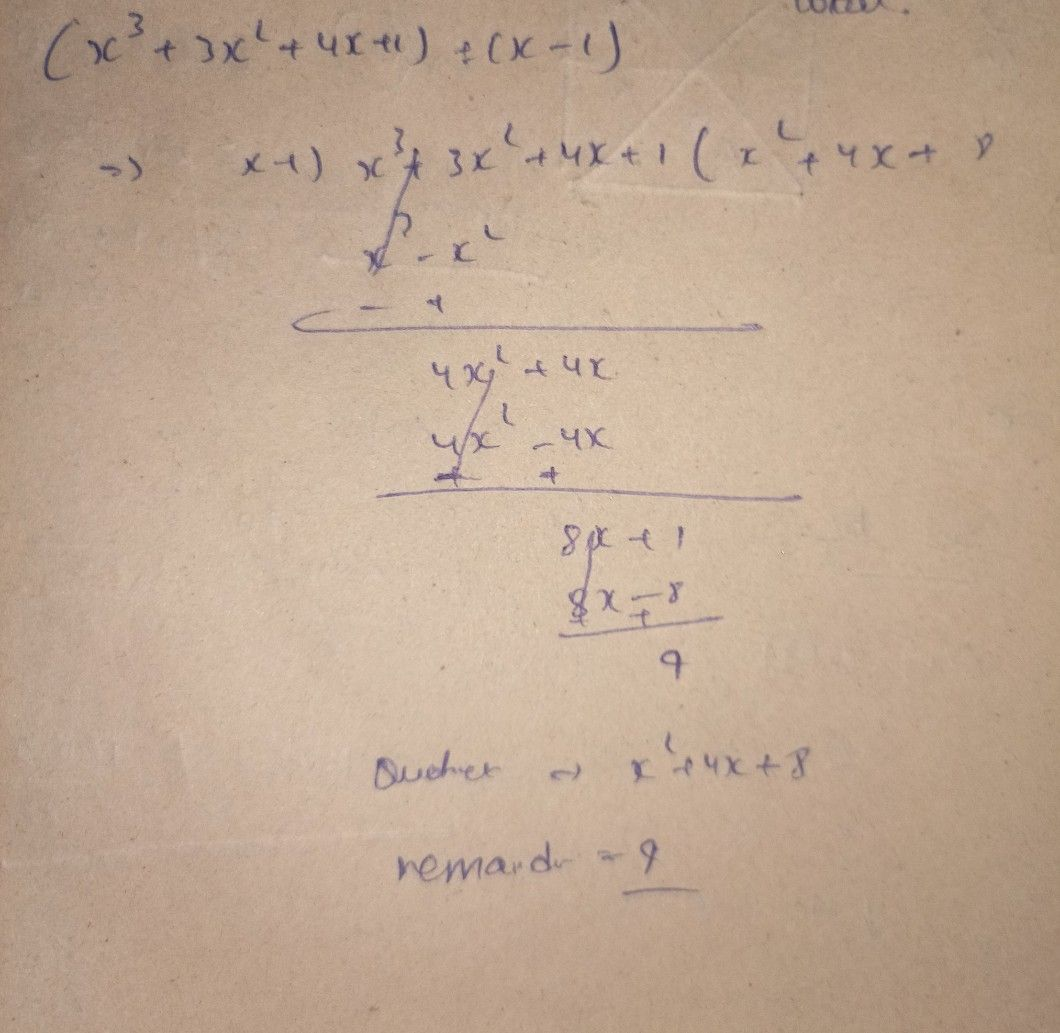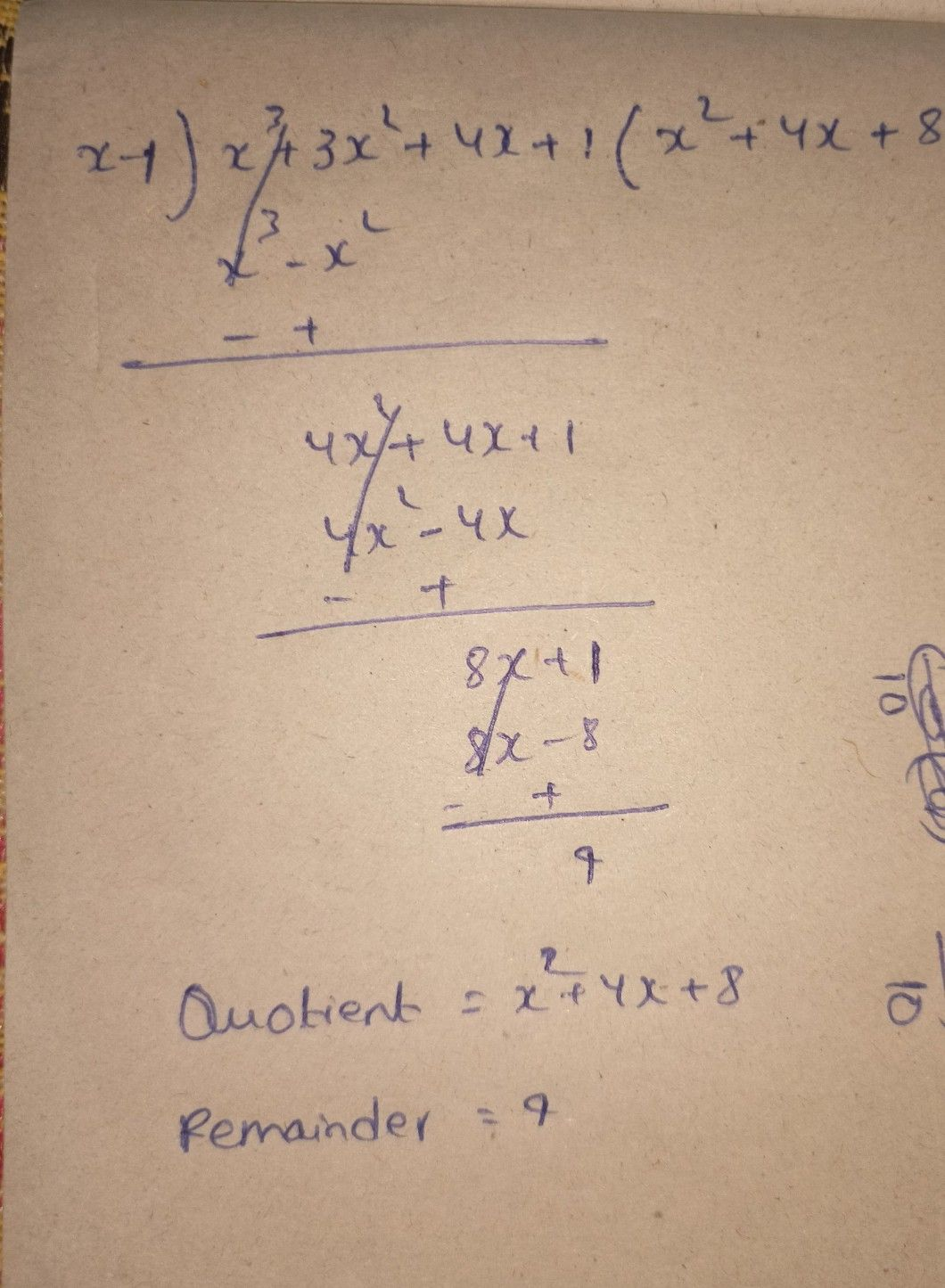Symbol
Problem$n$ Find the quotient and remainder $by$ uaing synthetic division. Write your complete solution on a separate sheet of paper. $Q006cat$ $2$ $\left(x^{2}+3x^{2}+4x+1\right)+\left(x-1\right)$ $cmaindc$
Other
SolutionQanda teacher - sundarStudent
I don't understand the numbers you've written can u write it againQanda teacher - sundar
1 minif you have any doubts ask meStudent
is the reminder 4 or 9Qanda teacher - sundar
it's 9
sorry for handwritingStudent
if I use long division will the answer be the same?
or will it changeQanda teacher - sundar
what
I didn't get you
what ever you did the answer
didn't change
answer must be equal for all methods
is there any queries?Student
nothing thanks for answering my queriesQanda teacher - sundar
if don't mine can get some extra coins